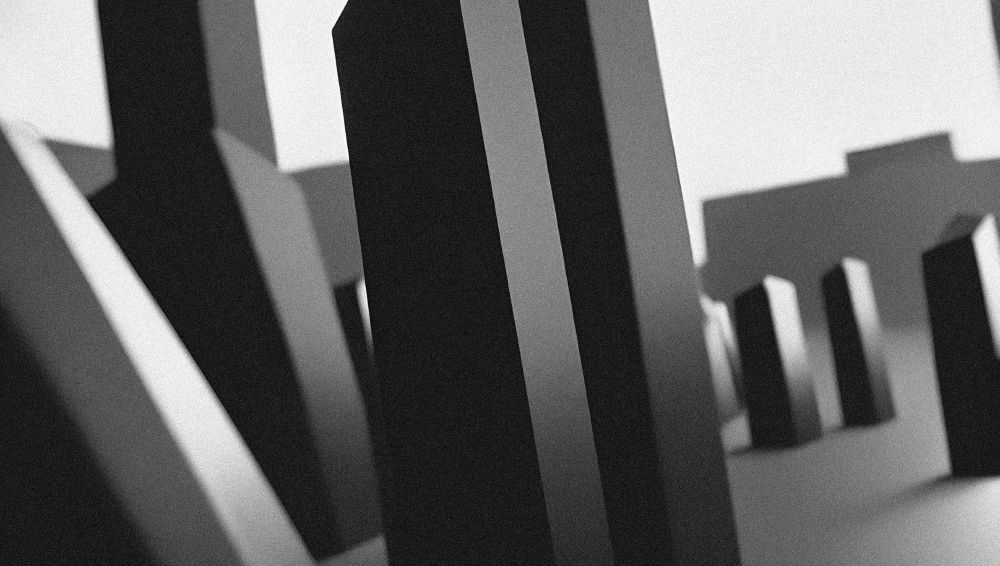# Space Mode

Space Mode is a multi-weighted typeface, sent back in time from the distant future.

Forward-looking typeface designers often predict a reductive future where Latin letterforms have become increasingly modularized and simplified, or random bits have mysteriously gone missing. Thankfully, this is not the case, and typography has instead flourished and evolved. New forms have appeared, and some revived from historical references. A more complex drawing model has arisen that seems to add new curves in a effort to tame the strange diagonals that appear in the final quarter of the alphabet.

## Features

• Light
• Regular
• Medium
• Semibold
• Bold
• Black

### Character set

• A
• B
• C
• D
• E
• F
• G
• H
• I
• J
• K
• L
• M
• N
• O
• P
• Q
• R
• S
• T
• U
• V
• W
• X
• Y
• Z

• a
• b
• c
• d
• e
• f
• g
• h
• i
• j
• k
• l
• m
• n
• o
• p
• q
• r
• s
• t
• u
• v
• w
• x
• y
• z

• À
• Á
• Â
• Ã
• Ä
• Å
• Ç
• È
• É
• Ê
• Ë
• Ì
• Í
• Î
• Ï
• Ñ
• Ò
• Ó
• Ô
• Õ
• Ö
• Ø
• Ù
• Ú
• Û
• Ü
• Ŵ
• Ý
• Ŷ
• Ÿ
• Æ
• Ð
• Þ
• Œ

• à
• á
• â
• ã
• ä
• å
• ç
• è
• é
• ê
• ë
• ì
• í
• î
• ï
• ı
• ȷ
• ñ
• ò
• ó
• ô
• õ
• ö
• ø
• ß
• ù
• ú
• û
• ü
• ŵ
• ý
• ÿ
• ŷ
• æ
• ð
• þ
• œ

• .
• ,
• :
• ;
• !
• ¡
• ?
• ¿
• ·
• *
• #
• /
• \
• -
• _
• (
• )
• {
• }
• [
• ]
• «
• »
• "
• '

• \$
• %
• +
• <
• =
• >
• ^
• ~
• £
• ×
• ÷

• 0
• 1
• 2
• 3
• 4
• 5
• 6
• 7
• 8
• 9

• @
• &
• §
• ®
• °
• |
• ¦

#### Diacritics

• ̈
• ̇
• ̀
• ́
• ̂
• ̊
• ̃
• ̧
• `
• ¨
• ´
• ¸
• ˆ
• ˙
• ˚
• ˜
• Extended Latin character set
• Six weights from Light to Black
• Unique mix of regular and descending capitals

Plasma Bionic

Global Tectonic

Psycho Robotic

## Get Space Mode

• Usage for desktop, web, app, ebook, social media, digital ads, and more!
• No need to track usage metrics (desktop users, web users, app downloads…)

6 styles

• Light
• Regular
• Medium
• Semibold
• Bold
• Black

### Trial Fonts

A fully-functioning trial version is available for testing and evaluation purposes. Students, teachers, and personal users can also use the trial fonts under a pay-what-you-can model.